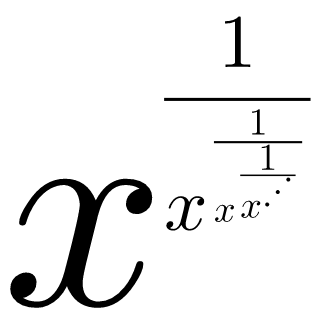# Real Numbers to Complex?!

Algebra Level 2Nest wrote a series of $x$'s in the fractional exponents He knows that if $x$ is a non-zero real number, then the equation on the right exists on the system of real numbers.

However, he thinks that it can also be a non-real complex number with $x$ being non-zero real number. Is it possible for this to happen?

×

Problem Loading...

Note Loading...

Set Loading...